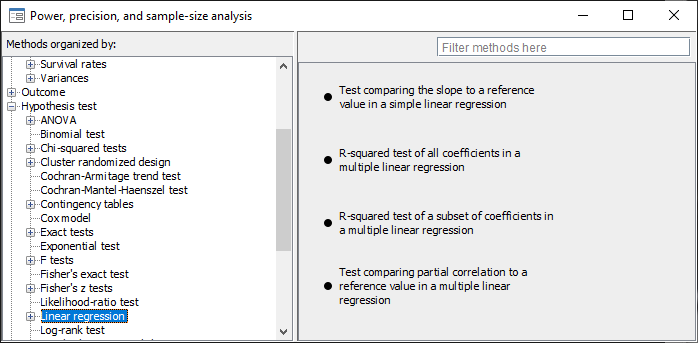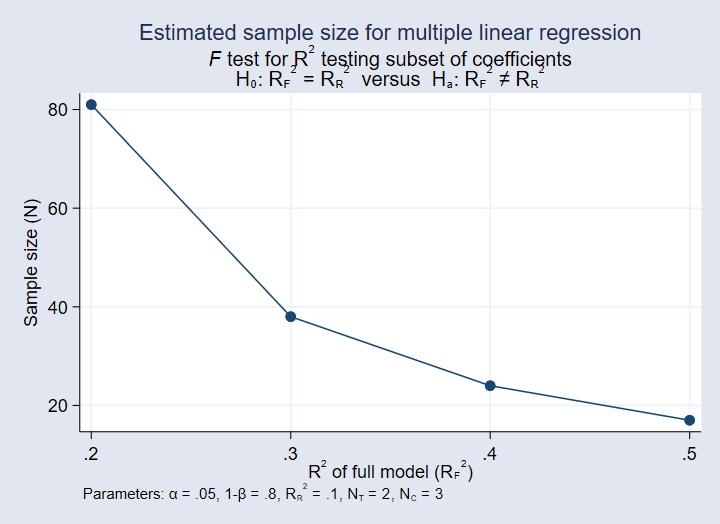»  Home »  Products »  Features »  Power analysis for linear regression

## Power analysis for linear regression

### Highlights

• Three methods for linear regression (LR)
• Slope test in a simple LR
• R2 test in a multiple LR
• Partial-correlation test in a multiple LR
• Multiple values of parameters
• Automatic and customizable tables
• Automatic and customizable graphs
See all features

Stata's power command performs power and sample-size analysis (PSS). Its features include PSS for linear regression.

As with all other power methods, the methods allow you to specify multiple values of parameters and to automatically produce tabular and graphical results.

### Linear regression

Stata's power command provides three PSS methods for linear regression.power oneslope performs PSS for a slope test in a simple linear regression. It computes one of the sample size, power, or target slope given the other two and other study parameters. See [PSS-2] power oneslope.

power rsquared performs PSS for an R2 test in a multiple linear regression. An R2 test is an F test for the coefficient of determination (R2). The test can be used to test the significance of all the coefficients, or it can be used to test a subset of them. In both cases, power rsquared computes one of the sample size, power, or target R2 given the other two and other study parameters. See [PSS-2] power rsquared.

power pcorr performs PSS for a partial-correlation test in a multiple linear regression. A partial-correlation test is an F test of the squared partial multiple correlation coefficient. The command computes one of the sample size, power, or target squared partial-correlation coefficient given the other two and other study parameters. See [PSS-2] power pcorr.

Here, we demonstrate PSS for an R2 test of a subset of coefficients in a multiple linear regression.

Consider a test of the significance of two covariates in a multiple linear regression adjusting for three other covariates. We will call the two covariates the tested covariates and the three others control covariates. The reduced model with the control covariates has an R2 of 0.1, and the full model with all five covariates has an R2 of 0.2. We want to compute the required sample size for the two-sided R2 test to achieve 80% power with a 5% significance level—power rsquared defaults.

. power rsquared 0.1 0.2, ntested(2) ncontrol(3)

Performing iteration ...

Estimated sample size for multiple linear regression
F test for R2 testing subset of coefficients
Ho: R2_F = R2_R  versus  Ha: R2_F != R2_R

Study parameters:

alpha =    0.0500
power =    0.8000
delta =    0.1250
R2_R =    0.1000
R2_F =    0.2000
R2_diff =    0.1000
ncontrol =         3
ntested =         2

Estimated sample size:

N =        81


We need 81 observations.

Suppose that we want to investigate the impact of the effect size on the required sample size. We plot below the sample-size curve as a function of the R2 values of the full model.

. power rsquared 0.1 (0.2(0.1)0.5), ntested(2) ncontrol(3) graphAs the R2 of the full model increases, the required sample size decreases. When the R2 is closer to 0.2, the curve is steeper.